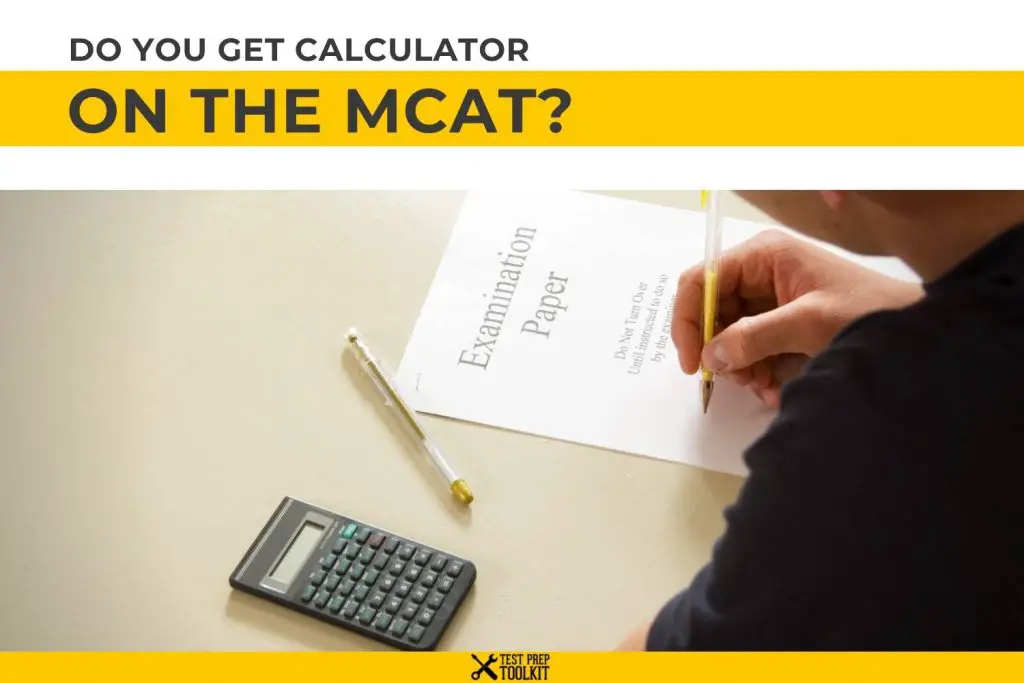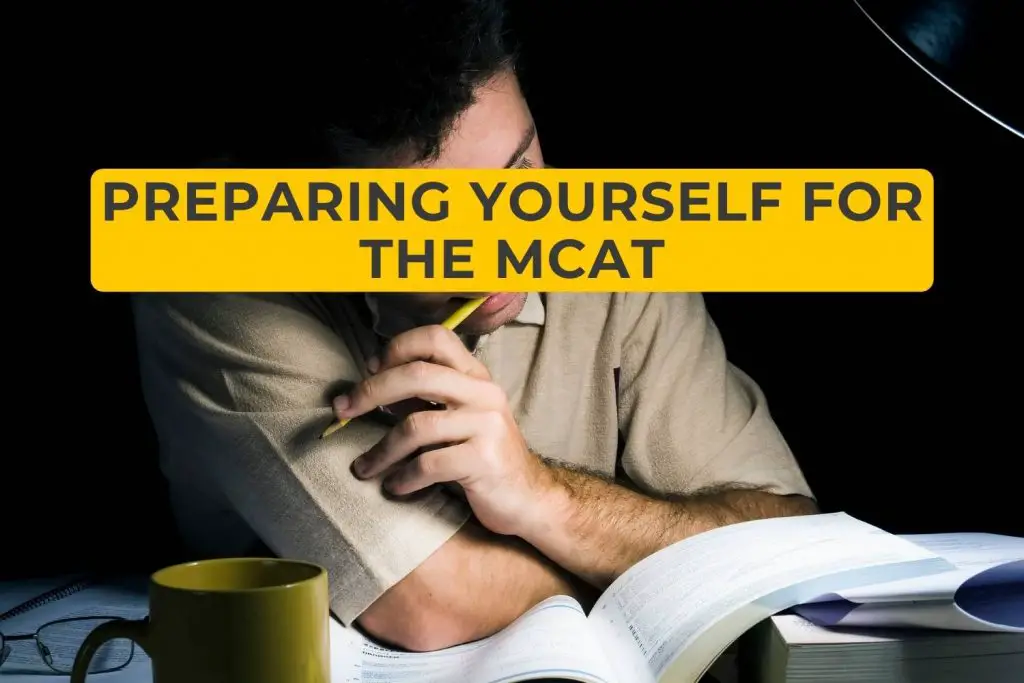# Do You Get a Calculator on the MCAT?

The medical school entrance exam, also known as the MCAT, finds many students diligently preparing in the weeks leading up to test day. As students are cramming and hoping for the best, it’s not uncommon for them to ask, “Do you get a calculator on the MCAT?”

With test day around the corner, you might feel confident that you know everything you need to know to pass the test: verbal and written reasoning skills, sciences, and a bit of math. But can you lean on a calculator during your test for the math portion of the exam?

Check out our post on the Best MCAT Prep Courses as well for you to know what’s best for you.

In this article, we’ll answer this question and explain what it means for you. We’ll share what that means for future test-takers and some tips to approach the MCAT when the time comes.

## Are You Allowed to Use a Calculator on the MCAT?Do you get a calculator on the MCAT? The short answer is no.

The AAMC does not allow test-takers to use a calculator while taking the test. The reason is that the MCAT is testing your mathematical problem-solving skills based on what you know about statistical reasoning, chemistry, physics, and more.

While you may wish you had access to a calculator, all the questions are of a design so that you don’t need one.

## Why Does the MCAT Not Allow Calculators?

Many people get hung up on the fact that the MCAT does not allow exam takers to use a calculator. But the reason is simple: the MCAT is not a mathematics test; it’s a test of reasoning.

The AAMC wants to see how you can work through a problem using logic, reason, and accumulated knowledge rather than how you can press buttons on a calculator.

The exam evaluates how well a student can read the question, analyze it, and approach the problem with rational thinking and logic. Knowing whether someone can type numbers into a calculator to get an appropriate number isn’t what they’re determining.

While it is not a mathematics test, some questions require strong mathematical thinking strategies and skills. One great example would be a question around acids and bases. Perhaps it asks the test-taker to identify the pH of a specific solution. To find this answer, the test taker would need to find a square root. It would be much easier with a calculator, but the point is to show your approach and how you arrive at the correct answer.

Ultimately, medical schools want to see that you can approach conceptual questions with reason and past knowledge. They want to see you demonstrate the practical and analytical reasoning you’ll need when practicing medicine.

## Preparing Yourself for the MCATIf you’re taking the MCAT and found yourself wondering, “Do you get a calculator on the MCAT?” don’t distress.

The best trick to overcoming a tricky math question without a calculator is leaning on mathematical strategies. You can apply these fundamentals to even the most complex seeming numbers.

The thing to know about the MCAT is that the test creators have designed the questions so that applicants can solve them using mental math and scratch work rather than a calculating machine. The key to finding the correct answer is thinking of the most straightforward and short way to arrive at the solution.

All the questions involve numbers that you can calculate by hand — in most cases, you can convert them into simpler figures such as decimals or fractions and work through them mentally.

If a student practices enough without a calculator, it should feel like second nature when you get to the actual testing center. You won’t even find yourself wishing you had a calculator.

You can also see our How Many Times Can You Take The MCAT for you to know more about the MCAT

## How to Study for the MCAT without a Calculator

Solving a bunch of math problems without a calculator by your side may seem daunting at first, but it won’t be as difficult as you imagine it. Keep in mind that you’ll be able to use scratch paper to see your work as you think it through, which is helpful for many.

If you’re still worried about handling these mental math problems, you’re best off practicing similar questions in the weeks leading up to the test. Don’t practice crazy equations — practice simple tricks and basic skills that you can use to manipulate any seemingly complex problem into a more digestible form.

Our article on How Long Does It Take to Study for the MCAT will surely help you on creating a study plan for the MCAT.

There are many test prep resources you can use to train with as you prepare for the exam. You can even utilize the MCAT Sample Question Guide through the AAMC. Also, it is very important to know When Should You Take The MCAT, so check our full post here. If you want to know how hard the MCAT is, see our post on How Hard Is The MCAT here.

## ConclusionThe biggest key to overcoming the mental barrier of not being able to use a calculator when taking the MCAT is practice. While you might be nervous now, you can get the hang of solving mathematical problems with reasoning and math tricks. You’ll come to realize that you can handle anything the test throws your way.